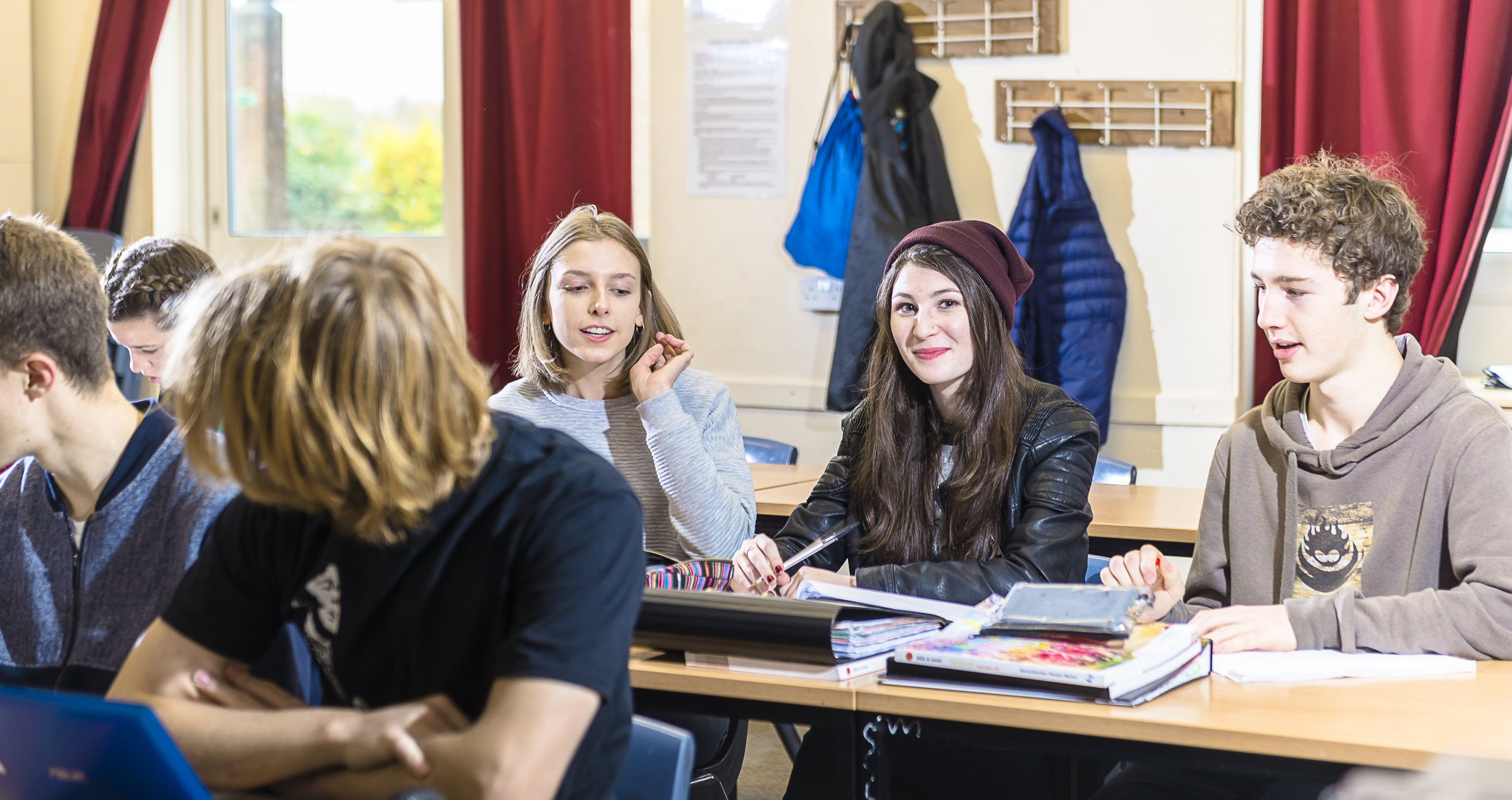### In This Section

Mathematics

Contact: gspurr@farmors.gloucs.sch.uk

### KS3 Mathematics

##### Course outline

During Key Stage 3 pupils receive 6 periods of Maths over two weeks. Pupils are placed in a broad setting at the beginning of year 7 based on their Key Stage 2 results. With the exception of the lowest set all pupils broadly follow the same curriculum during year 7 and 8 with extension being provided for the higher achievers in the form of questions designed to get them to apply their knowledge in context and problem solving situations. By the end of year 9 the vast majority of pupils will have covered the Mathematics Key Stage 3 National Curriculum. Progress is assessed during Key Stage 3 using topic based tracker tests, in class tests and the whole school assessments which every pupil will sit at various points through years 7 to 9.

##### Course Detail
Term 1 Term 2 Term 3 Term 4 Term 5 Term 6

7

Number – Number system factors, multiples and place value

Calculation with decimals and negatives

Introducing algebraic expressions

Initial setting week 2/3

Number – Fractions decimals and percentages, fraction calculations, powers/indices and ratio

Algebra -  Sequences and using formula

Geometry – Angle facts and reasoning

Statistics – Introducing probability

Mid-year Assessment

Algebra – Solving linear equations and plotting coordinates and lines

Geometry – Properties of shapes, perimeter, area, measurement  and scale drawing

Statistics – Recording and displaying data

End of Year Assessment

8

Number – Calculations and percentage change

Algebra – Expressions, solving linear equations

Geometry – Angles in parallel lines

Statistics – Venn Diagrams

Number – Fraction calculations, Using prime factor decomposition to find lowest common multiples and highest common factors and ratio problems

Algebra – Linear Sequences and relationships and more algebraic expressions including factorising

Geometry – Symmetry and construction

Statistics - Probability sample spaces and averages

Mid-year Assessment

Number – Estimation and accuracy

Algebra – Linear Equations, graphically and algebraically

Geometry – Area, perimeter and volume, Transformations including translation, rotation and reflection

Statistics  Recap

End of Year Assessment

9

Number – Index laws, Standard form, ratio and percentage change

Algebra – Geometric sequences, Expressions and formula,

Geometry – Angles in polygons

Statistics – Experimental probability and theoretical including frequency and probability trees

Number – Proportional reasoning

Algebra - Solving linear equations including fractions, straight line graphs and quadratic graphs

Geometry – Formula with geometry, Volume and surface area of prisms, Compound measures speed, density and pressure, Transformations including enlargement

Statistics – Collecting and representing  data including centrality and spread

Mid-year Assessment

Number – Revision of Number and ratio

Algebra – Using graphs to solve equations, solving basic quadratic equations, simultaneous equations

Geometry – Constructions and loci, Pythagoras and basic trigonometry

Statistics – Revision

End of Key Stage 3 Assessment

GCSE Foundation Paper

### KS4 Mathematics

Head of Key Stage 4 (GCSE): Graeme Spurr

Contact: gspurr@farmors.gloucs.sch.uk

Specification: Edexcel 1MA1

##### Course outline

The Mathematics GCSE covers a wide range of mathematical knowledge and skills with a strong focus on depth of understanding and problem solving. The knowledge and skills content is grouped into five areas:

• Number
• Algebra
• Ratio and Proportion
• Geometry
• Probability and Statistics

What should students expect to do during the course?

Students will be expected to:

• Recall and use standard mathematical skills and knowledge to solve problems.
• Be able to break down more complex problems into small steps in order to solve them.
• Be able to apply mathematical skills to analyse problems in a real life context.
• Be able to use a calculator to solve problems quickly and effectively.

Students will be given the opportunity to discuss mathematical ideas in groups and undertake investigations. The core textbooks and other resources develop learning through practical activities and traditional exercises. These books are supplemented by other appropriate materials, including computer software.

Homework is set on a regular basis to consolidate work taught in class or to revise topics previously learned. On-line homework may be set on occasions and students without internet access should speak to their teacher to get a hardcopy of the homework.

The assessment for the course consists of 3 papers all sat at the end of year 11.  Each paper is 1½ hours long and there is one non-calculator and two calculator papers.  Each paper is marked out of 80 giving a maximum total of 240 marks.

Students will be entered for either the foundation or higher tier exam papers. The higher tier examination is graded from 9 to 4 while the foundation tier is graded from 5 to 1.

##### Progression into Sixth Form

The GCSE prepares students to take any of the 3 maths options in the sixth form which include A level Mathematics; A level Further Mathematics and Core Mathematics.  The course also supports the subjects that require some level of maths which are the sciences, psychology, business & economics and geography

## A Level Core Maths